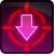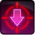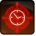# Champ atrophiqueConditions

## Used by

Used by
• Class 16140935293905669371

## Related effects, buffs and debuffs

Please click on an effect below to view its details.

• [hidden] [does not expire]Effect #1

 Slot: Debuff Duration: passive Tick rate: 1s # occurrences: 1
• When effect is applied
• When effect ticks

Only when the following conditions are met:

• If PRIMARY TARGET does not have 10 or more effects with the tag tag.​abl.​operation.​iokath.​boss.​scyva.​adds.​stasis_swarm_droid.​atrophic_field

Perform the following actions:

• Add effect #2 to TARGET from TARGET
• On Apply

Perform the following actions:

• Play appearance epp . operation . iokath . boss . scyva . adds . stasis_swarm_droid . atrophic_field, dependent on calling effect
• On Apply

Only when the following conditions are met:

• If difficulty is set to Story Mode

Perform the following actions:

• Set movement speed to 70%
• On Apply

Only when the following conditions are met:

• If difficulty is set to Hard or Nightmare Mode

Perform the following actions:

• Set movement speed to 30%
• [6s]Atrophie
Vitesse de déplacement et alacrité réduites.

 Slot: Debuff Duration: 6s Tick rate: does not tick Initial stacks: 1 Max stacks: 10 # occurrences: 1 Tags: tag.​abl.​operation.​iokath.​boss.​scyva.​adds.​stasis_swarm_droid.​atrophic_field Conditions: Can only by called by other effects
• When effect is applied
• When stacks of this effect increase

Only when the following conditions are met:

• If difficulty is set to Story Mode

Perform the following actions:

• When effect is applied
• When stacks of this effect increase

Only when the following conditions are met:

• If difficulty is set to Hard or Nightmare Mode

Perform the following actions:

• When stacks of this effect increase
• [target override]

Only when the following conditions are met:

• If stacks of this effect = 10

Perform the following actions:

• Add effect #3 to TARGET from TARGET
• When stacks of this effect increase
• When effect is applied

Only when the following conditions are met:

• If difficulty is set to 8-man SM

Perform the following actions:

• Spell Damage
- Unknown (609) = (bool) false
- Damage Type = (int) 2
- Slot = (int) 1
- Spell Type = (int) 2
- Level Cap = (int) 0
- Amount Max = (float) 0
- Amount Min = (float) 0
- Amount Percent = (float) 0
- Threat Percent = (float) 0
- Standard Health Percent Max = (float) 0.02
- Standard Health Percent Min = (float) 0.02
- Amount Modifier Percent = (float) 0
- Coefficient = (float) 0
- Health Steal Percentage = (float) 0
• When stacks of this effect increase
• When effect is applied

Only when the following conditions are met:

• If difficulty is set to 16-man SM

Perform the following actions:

• Spell Damage
- Unknown (609) = (bool) false
- Damage Type = (int) 2
- Slot = (int) 1
- Spell Type = (int) 2
- Level Cap = (int) 0
- Amount Max = (float) 0
- Amount Min = (float) 0
- Amount Percent = (float) 0
- Threat Percent = (float) 0
- Standard Health Percent Max = (float) 0.03
- Standard Health Percent Min = (float) 0.03
- Amount Modifier Percent = (float) 0
- Coefficient = (float) 0
- Health Steal Percentage = (float) 0
• When stacks of this effect increase
• When effect is applied

Only when the following conditions are met:

• <
• If difficulty is not set to Nightmare Mode
AND
• If difficulty is set to 8-man HM
>

Perform the following actions:

• Spell Damage
- Unknown (609) = (bool) false
- Damage Type = (int) 2
- Slot = (int) 1
- Spell Type = (int) 2
- Level Cap = (int) 0
- Amount Max = (float) 0
- Amount Min = (float) 0
- Amount Percent = (float) 0
- Threat Percent = (float) 0
- Standard Health Percent Max = (float) 0.02
- Standard Health Percent Min = (float) 0.02
- Amount Modifier Percent = (float) 0
- Coefficient = (float) 0
- Health Steal Percentage = (float) 0
• When stacks of this effect increase
• When effect is applied

Only when the following conditions are met:

• <
• If difficulty is not set to Nightmare Mode
AND
• If difficulty is set to 16-man HM
>

Perform the following actions:

• Spell Damage
- Unknown (609) = (bool) false
- Damage Type = (int) 2
- Slot = (int) 1
- Spell Type = (int) 2
- Level Cap = (int) 0
- Amount Max = (float) 0
- Amount Min = (float) 0
- Amount Percent = (float) 0
- Threat Percent = (float) 0
- Standard Health Percent Max = (float) 0.04
- Standard Health Percent Min = (float) 0.04
- Amount Modifier Percent = (float) 0
- Coefficient = (float) 0
- Health Steal Percentage = (float) 0
• When stacks of this effect increase
• When effect is applied

Only when the following conditions are met:

• <
• If difficulty is set to Nightmare Mode
AND
• If difficulty is set to 8-man HM
>

Perform the following actions:

• Spell Damage
- Unknown (609) = (bool) false
- Damage Type = (int) 2
- Slot = (int) 1
- Spell Type = (int) 2
- Level Cap = (int) 0
- Amount Max = (float) 0
- Amount Min = (float) 0
- Amount Percent = (float) 0
- Threat Percent = (float) 0
- Standard Health Percent Max = (float) 0.04
- Standard Health Percent Min = (float) 0.04
- Amount Modifier Percent = (float) 0
- Coefficient = (float) 0
- Health Steal Percentage = (float) 0
• When stacks of this effect increase
• When effect is applied

Only when the following conditions are met:

• <
• If difficulty is set to Nightmare Mode
AND
• If difficulty is set to 16-man HM
>

Perform the following actions:

• Spell Damage
- Unknown (609) = (bool) false
- Damage Type = (int) 2
- Slot = (int) 1
- Spell Type = (int) 2
- Level Cap = (int) 0
- Amount Max = (float) 0
- Amount Min = (float) 0
- Amount Percent = (float) 0
- Threat Percent = (float) 0
- Standard Health Percent Max = (float) 0.05
- Standard Health Percent Min = (float) 0.05
- Amount Modifier Percent = (float) 0
- Coefficient = (float) 0
- Health Steal Percentage = (float) 0
• [6s]Synchronisation terminée
En attente du destin.

 Slot: Buff Duration: 6s Tick rate: does not tick # occurrences: 1 Tags: tag.​abl.​buff.​interrupt_immunity, tag.​abl.​operation.​iokath.​boss.​scyva.​adds.​stasis_swarm_droid.​synchronization_complete Conditions: Can only by called by other effects
• On Apply

Perform the following actions:

• Unknown (165)
- Attack Wave = (int) 2
• Grant Immunity
- Ability Spec = (int) 0
- Damage Type List = Int(5)
- Damage Channel List = Int(1)
• When effect expires

Perform the following actions:

• Unknown (165)
- Attack Wave = (int) 1